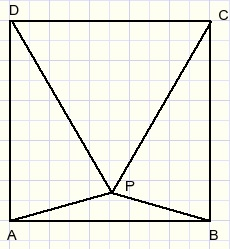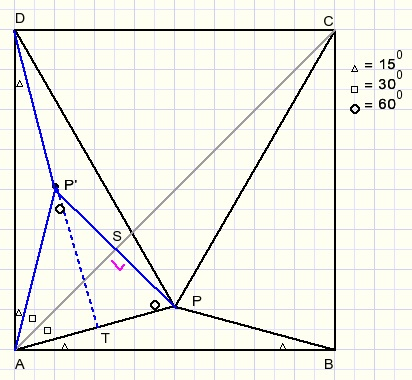Geometry problemPlease look at the next geometry problem:Given is square ABCD.
Triangle CDP is equilateral.
Calculate the size of LBAP.

This calculation is not difficult. The result is 150.

However, the reverse problem is not so easy:
Given is square ABCD and point P within such that LPAB = LPBA = 150.
Prove that triangle CDP is equilateral.

How to start such a proof?
The 150 angle does not bring us far.
We have to use the fact that ABCD is a square.
So let's start by reflecting ABP around diagonal AC.
Now look at the next picture, somewhat enlarged for clarity:Point P' is the reflection of P on line AC.
Now focus on triangle APP'.
AP = AP' and LPAP' = 90 - 2*15 = 600.
LAPP'= (180 - 60)/2 = 600 so triangle APP' is equilateral.

We extend line DP' to point T on AP.
LATD = 180-15-15-60 = 900
P'T is perpendicular to AP and because triangle APP' is equilateral, T is the center of AP.
DT is the perpendicular bisector of AP.
So DA = DP.
For reasons of symmetry CP = DP.
Because ABCD is a square AD = CD.
Triangle CDP is equilateral.

There may be better, shorter of more creative solutions.
Please let me know and I will be happy to publish your solution below.OneStat